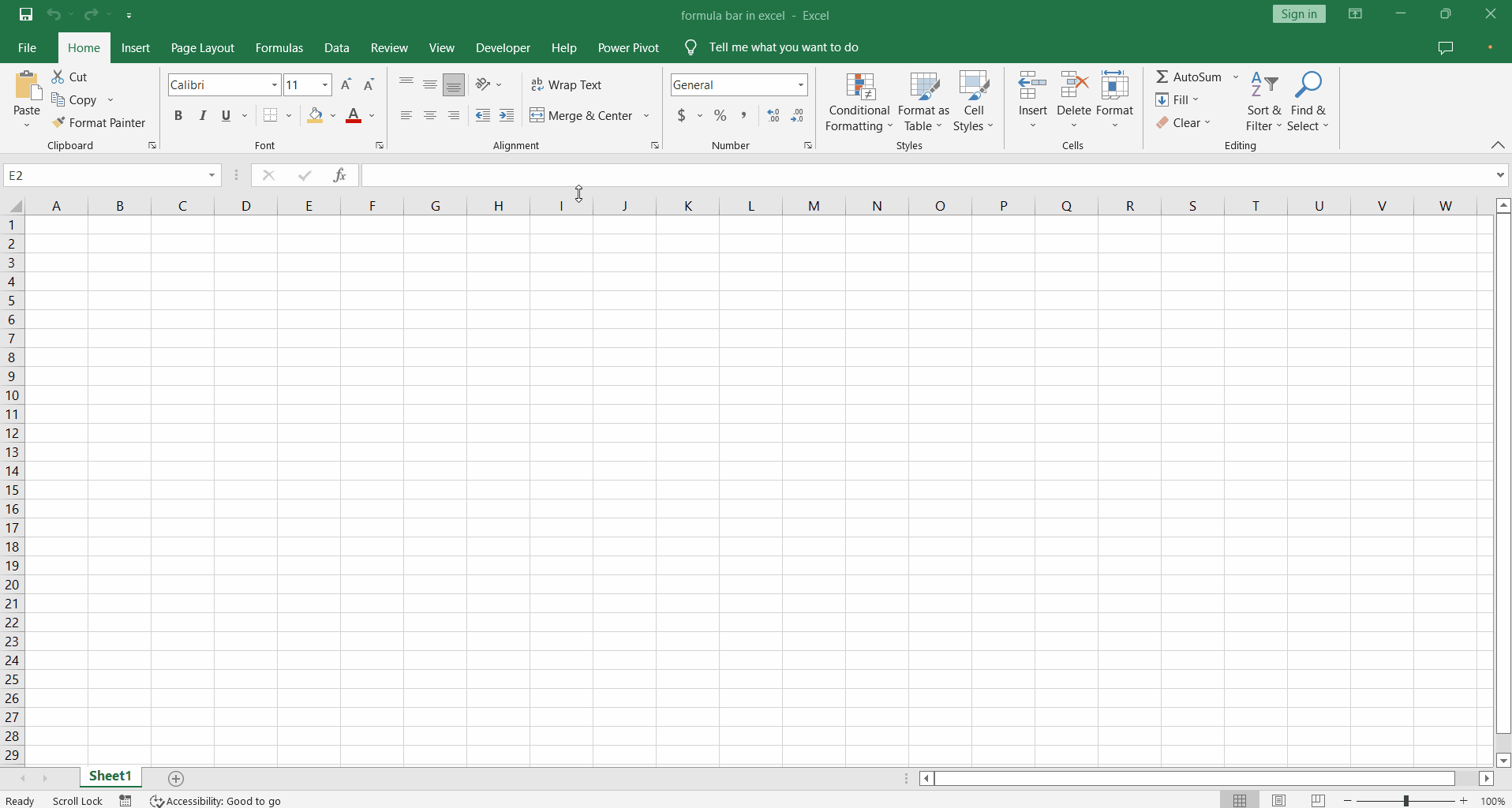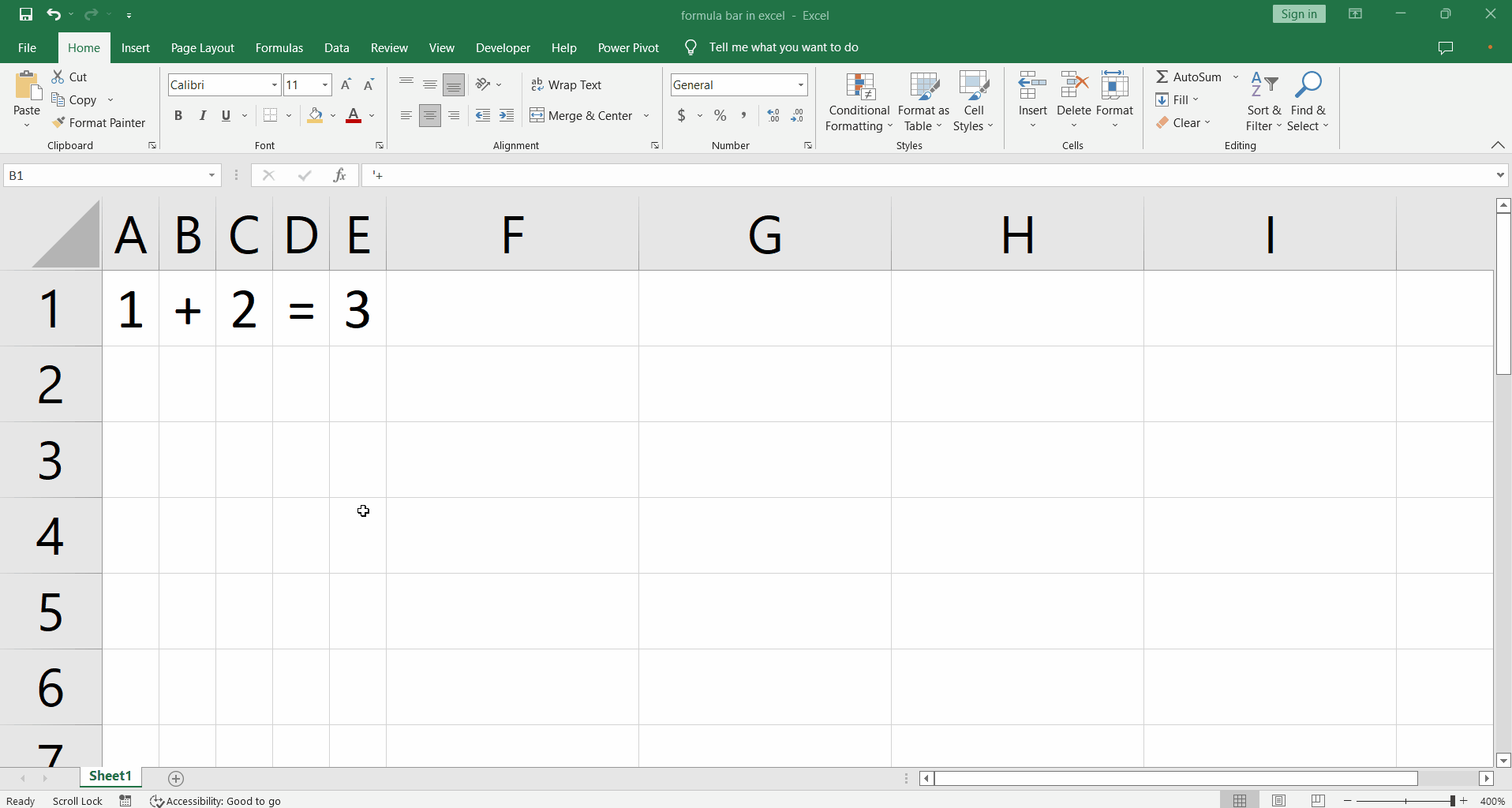# How to Enable and use formula bar in excelThe formula bar is one of the most important toolbar located at the top of the excel worksheet. It allows formula inputs for the calculations to be carried out in the excel workbooks. Let’s know more about formula bars.

### Step 1 – Show / Hide formula bar– In order to show or hide the formula bar go to the View tab in excel and click the checkbox next to the Formula Bar option in the Show group.

### Step 2 – Expand / Contract formula bar– To expand or contract the formula bar hover the mouse at the lower end of the bar till it changes to an up-and-down arrow.

– Click and drag up or down to contract or expand.

– This allows us to view long formulas completely within the formula bar.

### Step 3 – Write formulas in formula bar– So the actual purpose of the formula bar is to write all sorts of formulas that come with excel.

– Whenever a cell is selected we can see the formula for that cell in the formula bar.